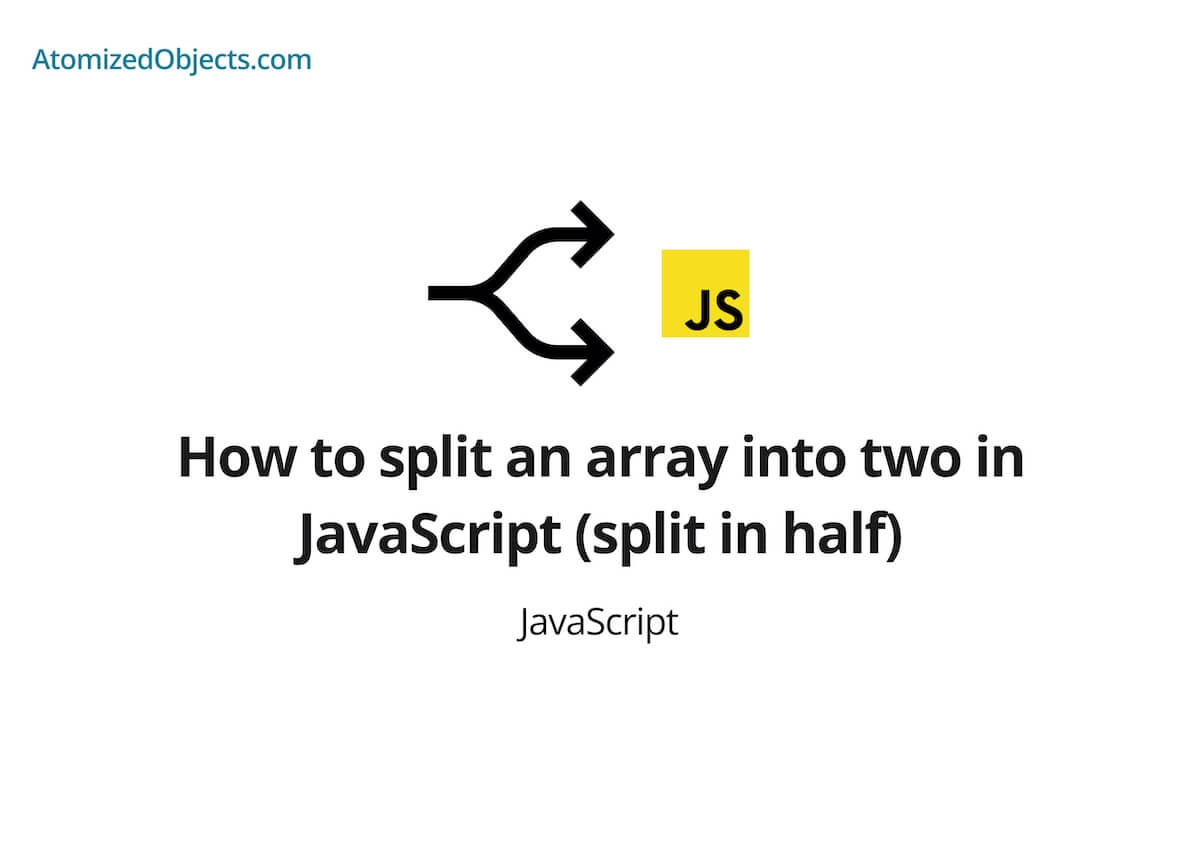Blog / Javascript

# How to split an array into two in JavaScript (split in half)In this short post we go over everything you need to know to split an array into two in javascript including in half and by valueWill Mayger
August 20, 2021
Article

In this post we will be covering how to split an array into two in JavaScript (split in half) with as little technical jargon as possible so you will have everything you need right here without having to look any further!

Splitting an array into two in JavaScript is actually really easy to do using the Array.prototype.slice method.

The Array.prototype.slice method lets us create a new array based on the indexes we provide it and is how we are going to split an array into two.

## How to split an array into two in JavaScript

For this first solution we are going to look into dividing an array in half in JavaScript so that we are left with two arrays both of which have half the length of the first.

To do this we are going to use the Array.prototype.slice method, here is how it will looks:

``````const myArray = [1, 2, 3, 4]

const halfWayIndex = Math.ceil(myArray.length / 2)

const firstHalfOfArray = myArray.slice(0, halfWayIndex)
const secondHalfOfArray = myArray.slice(halfWayIndex)

console.log(firstHalfOfArray) // [1, 2]
console.log(secondHalfOfArray) // [3, 4]``````

As you can see, we have now split an array into two in JavaScript (split in half) with the Array.prototype.slice method.

We do this by first finding the index that is half way through the array, once we have that index we know that we need to get all items up to that point and save that into a new array, and then do the same for all items after that index as well for the second half.

You might be wondering what the `Math.ceil` is for.

We are using `Math.ceil` here in case we have an odd length of our array, in the example above we have 4 items so the length is even which gives us a whole number or an integer when we divide the length in half.

If the length was an odd number, for example 5, when we divide it in half we will end up with a decimal or a float of 2.5.

Where indexes use whole numbers this would be an issue so we need to then either round up or down to get a whole number.

Here is an example of splitting an array into two that is odd sized:

``````const myArray = [1, 2, 3, 4, 5]

const halfWayIndex = Math.ceil(myArray.length / 2)

const firstHalfOfArray = myArray.slice(0, halfWayIndex)
const secondHalfOfArray = myArray.slice(halfWayIndex)

console.log(firstHalfOfArray) // [1, 2, 3]
console.log(secondHalfOfArray) // [4, 5]``````

This method works really well if you are wanting to divide an array into equal parts in JavaScript, but you can also use it to divide it into any two different sizes you want by changing the indexes.

### How to split an array into multiple arrays based on a value in JavaScript

The next thing to look at is if you want to split an array into multiple arrays based on a value.

It is actually pretty easy to do and very similar to the first two examples except instead of finding the halfway point in an array, we need to find a value within the array.

To do this we can use Array.prototype.indexOf to find the index of a value and then we can pass that into the slice method to split an array in two.

Here is how we can do this using Array.prototype.indexOf in JavaScript:

``````const myArray = ["a", "b", "c", "d", "e"]

const valueToSplitAt = myArray.indexOf("d")

const firstPartOfArray = myArray.slice(0, valueToSplitAt)
const secondPartOfArray = myArray.slice(valueToSplitAt)

console.log(firstPartOfArray) // ['a', 'b', 'c']
console.log(secondPartOfArray) // ['d', 'e']``````

And if you instead want to split the array by that value and not include the value in either of the arrays, we just need to skip over that index using some addition like so:

``````const myArray = ["a", "b", "c", "d", "e"]

const valueToSplitAt = myArray.indexOf("d")

const firstPartOfArray = myArray.slice(0, valueToSplitAt)
const secondPartOfArray = myArray.slice(valueToSplitAt + 1)

console.log(firstPartOfArray) // ['a', 'b', 'c']
console.log(secondPartOfArray) // ['e']``````

### Summary

There we have how to split an array into two in JavaScript (split in half), if you want more like this be sure to check out some of my other posts!Foxi

Budget Planner & Tracker

More money in your pocket by the end of the month.

Free to use and no account needed.

Get started now.

Some graphics used on this post were made using icons from flaticon.